Faculty of Physics
M.V. Lomonosov Moscow State University

# Issue 1, 2017

Theoretical and mathematical physics

## Mathematical Modeling of Randomness and Fuzziness Phenomena in Scientific Studies: I. Mathematical and Empirical Foundations## Mathematical Modeling of Randomness and Fuzziness Phenomena in Scientific Studies: I. Mathematical and Empirical Foundations

### Yu. P. Pyt'ev

Moscow University Physics Bulletin 2017. 72. N 1. P. 1

The possibility theory as a mathematical model of randomness and fuzziness phenomena is considered in a variant that enables the modeling of both probabilistic randomness, including that inherent in unpredictably evolving stochastic objects whose probabilistic models cannot be empirically reconstructed and nonprobabilistic randomness (fuzziness) inherent in real physical, technical, and economical objects, human–machine and expert systems, etc. Some principal distinctions between the considered variant and the known possibility theory variants, in particular, in mathematical formalism and its relationship with probability theory, substantive interpretation, and applications exemplified by solving the problems of identification and estimation optimization, empirical recostruction of a fuzzy model for a studied object, measurement data analysis and interpretation, etc. (in the paper “Mathematical Modeling of Randomness and Fuzziness Phenomena in Scientific Studies. II. Applications”) are shown.

Show Abstract
Theoretical and mathematical physics

## The quasi-exactly solvable problems for two dimensional quantum systems

### Liyan Liu$^1$, Chong Hou$^2$, Liqian Wei$^3$

Moscow University Physics Bulletin 2017. 72. N 1. P. 36

In this paper, we study the quasi-exactly solvable problems for two dimensional quantum systems. By using the Bethe ansatz method, we obtain the general form of the quasi-exactly solvable potential. Then, we present several examples to give the specific forms of quasi-exactly solvable potentials. In the examples, some physical models of quasi-exactly solvable problems are re-exhibited.

Show Abstract

## A Hybrid Method for the Solution of the Poisson Equation in the Domain of Metal-Dielectric Corners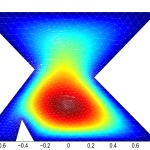## A Hybrid Method for the Solution of the Poisson Equation in the Domain of Metal-Dielectric Corners

### A.N. Bogolyubov, A.I. Erokhin, I.E. Mogilevsky, M.I. Svetkin

Moscow University Physics Bulletin 2017. 72. N 1. P. 16

The Poisson’s equation in a two-dimensional domain that contains star metal-dielectric corners is considered. The corners can be only metal or only dielectric. In their neighborhood, the solution is bounded, but the gradient has power-law singularities. A numerical algorithm for solving this problem is proposed. The algorithm is based on the finite-element method and takes the asymptotic behavior of the solution in the neighborhood of metal-dielectric corners into account.

Show Abstract

## A New Method to Obtain the Carnahan–Starling Equation and Its Generalization## A New Method to Obtain the Carnahan–Starling Equation and Its Generalization

### P.N.Nikolaev

Moscow University Physics Bulletin 2017. 72. N 1. P. 23

We obtain the Carnahan–Starling equation for a system of hard spheres using the Euler method of accelerated series convergence. For this purpose, the virial series is transformed into a new series with coefficients that differ slightly from each other, even when considering the eleven currently known virial coefficients. The method of accelerated convergence was applied to this series; it allowed us to obtain the Carnahan– Starling equation. In this work, this equation is derived for the first time using the method of accelerated convergence. It is generalized to accurately reproduce all of the known virial coefficients and the asymptotic behavior of the free energy at high densities. This also makes it possible to describe a metastable region with a high degree of accuracy and to obtain the equation of state for a homogeneous system of hard spheres with the accuracy of a computer experiment.

Show Abstract

## Confinement of Atoms with Robin’s Condition: Spontaneous Symmetry Breaking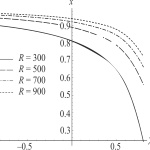## Confinement of Atoms with Robin’s Condition: Spontaneous Symmetry Breaking

### K.A. Sveshnikov$^1$, P.K. Silaev$^2$, A.V. Tolokonnikov$^2$

Moscow University Physics Bulletin 2017. 72. N 1. P. 29

The properties of the ground state of the hydrogen atom in a spherical vacuum cavity with general boundary “not going out” conditions (i.e., when the probability current through the boundary vanishes) are studied. It is shown that in contrast to the confinement of an atom by a potential barrier, in this case depending on the parameters of the cavity, the atom could be in stable equilibrium at the center of the cavity or shift towards its periphery: spontaneous breaking of spherical symmetry occurs. The phase diagram of the shift and the dependence of the shift value and the binding energy of the ground state of the atom on the cavity parameters are presented. At the same time, the deformation properties of the electron wave function (WF) for an asymmetric distortion are so nontrivial that a non-zero shift occurs even when an electron is repulsed from the cavity boundary.

Show Abstract
Physics of nuclei and elementary particles

## The Spins of Induced-Fission Fragments within the Dynamic Approach## The Spins of Induced-Fission Fragments within the Dynamic Approach

### D.O. Eremenko$^{1,3}$, V.A. Drozdov$^3$, S.Yu. Platonov$^{1,3}$, O.V. Fotina$^{2,3}$, O.A. Yuminov$^3$

Moscow University Physics Bulletin 2017. 72. N 1. P. 39

A new approach to calculating the mean spins of induced-fission fragments is proposed. This approach, which has the key dynamic aspects of induced fission factored in, was used to analyze experimental data on the angular and energy dependences of mean spins and on the anisotropy of the angular distributions of fission fragments for the 16O + 232Th reaction at Ecm = 80–150 MeV. The range of relaxation times that provide a consistent description of the experimental data was determined for the degree of freedom associated with the orientation of the symmetry axis of a fissioning nucleus with respect to the total angular momentum.

Show Abstract

## The Characteristics of the Grazing Interaction of Fast Electrons with Dielectric Surfaces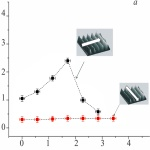## The Characteristics of the Grazing Interaction of Fast Electrons with Dielectric Surfaces

### K.A. Vokhmyanina$^1$, A.A. Kaplii$^1$, A.S. Kubankin$^{1,2}$, V.S. Sotnikova$^1$, T.B. Nikulicheva$^1$, A.S. Chepurnov$^3$

Moscow University Physics Bulletin 2017. 72. N 1. P. 45

Experimental data on the mechanism of the grazing interaction of fast electron beams with planar dielectric surfaces of various materials are analyzed. New experimental results regarding the grazing interaction between electrons with an energy of 10 keV and metalized structured surfaces are reported. The difference between the processes of the interaction of fast electron beams with planar and structured surfaces is demonstrated

Show Abstract

## The Single-Particle Characteristics of Pb Isotopes Near the Drip Lines Calculated within the Dispersive Optical Model## The Single-Particle Characteristics of Pb Isotopes Near the Drip Lines Calculated within the Dispersive Optical Model

### O.V. Bespalova$^1$, A.A. Klimochkina$^1$, A.V. Korotkov$^2$, T.I. Spasskaya$^1$

Moscow University Physics Bulletin 2017. 72. N 1. P. 51

The neutron and proton dispersive optical potential for the 208Pb nucleus has been determined for the energy region from –70 to +60 MeV and used to calculate the differential elastic scattering, the total interaction and reaction cross sections, as well as the single-particle characteristics, the neutron and charge densities, rms radii, and the thickness of the nucleus skin. The calculated results are in good agreement with the experimental data. The proton dispersive optical model potential for the spherical and close to spherical Pb isotopes within the neutron and proton drip lines has been obtained by a similar method. The calculation predicts a trend towards the growth of the proton particle-hole gap, which corresponds to Z = 82 shell closure as Z approaches the proton drip line.

Show Abstract

## Generation of a Vortex Ultrasonic Beam with a Phase Plate with an Angular Dependence of the Thickness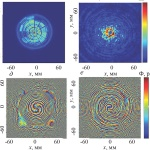## Generation of a Vortex Ultrasonic Beam with a Phase Plate with an Angular Dependence of the Thickness

### M.E. Terzi, S.A. Tsysar, P.V. Yuldashev, M.M. Karzova, O.A. Sapozhnikov

Moscow University Physics Bulletin 2017. 72. N 1. P. 61

Vortex-wave beams are beams that carry angular momentum. Their specific feature is a ring-like transverse distribution of wave intensity with zero intensity at the axis. A method for generating an ultrasonic vortex beam by combining a single-element transducer and a phase plate with a nonuniform thickness is proposed. The method is examined theoretically and tested experimentally. In the theoretical analysis, the acoustic field was calculated using the Rayleigh integral. Experiments were performed in water with a focusing piezoceramic source with a frequency of the order of 1 MHz; the radiation from it was transmitted through a 12-sector organic-glass phase plate. The beam vorticity was established by setting the correct thickness of sectors. The results of scanning the field with a miniature hydrophone confirmed that the amplitude and phase distributions of the generated wave field were in fact consistent with a vortex beam. The capacity of the obtained beam to induce the rotation of scatterers positioned in the focal region was demonstrated.

Show Abstract
Optics and spectroscopy. Laser physics

## An Analysis of Dissolved Organic Matter from Freshwater Karelian Lakes Using Reversed-Phase High-Performance Liquid Chromatography with Online Absorbance and Fluorescence Analysis online absorbance and fluorescence detection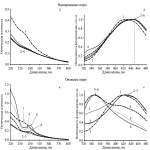## An Analysis of Dissolved Organic Matter from Freshwater Karelian Lakes Using Reversed-Phase High-Performance Liquid Chromatography with Online Absorbance and Fluorescence Analysis online absorbance and fluorescence detection

### D.A. Khundzhua$^1$, S.V. Patsaeva$^1$, O.A. Trubetskoj$^2$, O.E. Trubetskaya$^3$

Moscow University Physics Bulletin 2017. 72. N 1. P. 68

The spectral and optical properties of the fractionated components of dissolved organic matter (DOM) of three freshwater lakes in Karelia were studied using reversed-phase high-performance liquid chromatography (RP-HPLC) with online detection of fluorescence and absorption spectra. It is shown that the DOM fractions are qualitatively similar, but differ quantitatively in the ratio of components and consist of at least three types of fluorophores: (1) hydrophilic “humic-like” fluorophore(s) with the emission maximum in the region of 420 nm and an absorption band at 260–270 nm; (2) hydrophobic “humic-like” fluorophore(s) with the emission maximum at approximately 450 nm that has no characteristic absorption maxima in the region from 220 to 400 nm; and (3) a “protein-like” fluorophore with the emission maximum in the region of 340–350 nm, which is typical of proteins and peptides containing tryptophan

Show Abstract

## On the Possibility of an Incorrect Choice of a Quantization Volume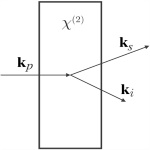## On the Possibility of an Incorrect Choice of a Quantization Volume

### Belinsky A.V.

Moscow University Physics Bulletin 2017. 72. N 1. P. 76

The specific features of the choice of an incorrect quantization volume, based on the example of the preparation of a multimode quantum state in parametric scattering, are considered. A small definitely incorrect volume that substantially simplifies the solution of the problem is chosen; it is shown to yield correct results except for an insignificant phase factor, which is proved by comparison with a more accurate correct (in the sense of the quantization volume) solution. This provides hope that the solution of quantum problems can be considerably simplified, at least to a first approximation

Show Abstract
Condensed matter physics

## Investigation of Energy Losses in Low-Coercivity Resin-Bonded Magnets in Alternating Magnetic Fields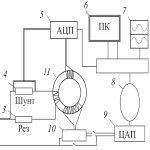## Investigation of Energy Losses in Low-Coercivity Resin-Bonded Magnets in Alternating Magnetic Fields

### E.V. Milov, I.A.Sipin, V.N. Milov, A.S.Andreenko, I.A.Balan

Moscow University Physics Bulletin 2017. 72. N 1. P. 80

Energy losses during alternating remagnetization of low-coercitivity resin-bonded magnets and commercially produced electrical steels were studied experimentally. The studies were conducted on several samples of resin-bonded magnets with different manufacturing technologies and samples of electrical steel sheets of various thicknesses. The static and dynamic magnetic properties of the samples were measured on a vibration magnetometer and a specially designed apparatus, respectively. It was found that the studied samples of bonded magnets have a relatively high level of hysteresis losses associated with high coercivity, which reaches a value of 4–5 Oe. At the same time, the remagnetization losses due to the Foucault currents in the bonded magnets are considerably lower than in electrical steels. The measurement results show that bonded magnets at high frequencies of remagnetization, especially in high-rpm motors, can be competitive in comparison with electrical steels.

Show Abstract

## The Interaction between Polyelectrolyte Microgels of N-Isopropylacrylamide Copolymers and Aqueous Solutions of Alcian Blue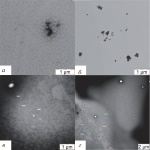## The Interaction between Polyelectrolyte Microgels of N-Isopropylacrylamide Copolymers and Aqueous Solutions of Alcian Blue

### D. V. Bogdashkina$^1$, K. E. Dashyan$^1$, E. E. Makhaeva$^1$, S. S. Abramchuk$^2$

Moscow University Physics Bulletin 2017. 72. N 1. P. 88

The temperature influence on the behavior of microgels based on poly-N-isopropylacrylamide (PNIPA) homopolymer, N-isopropylacrylamide copolymers with sodium methacrylate (PNIPA/MANa), sodium styrenesulfonate (PNIPA/SSNa), and sodium vinylsulfonate (PNIPA/VSNa) was studied. The comparative analysis of the temperature-induced collapse of macro- and microgels was conducted. The interaction between alcian blue and PNIPA, PNIPA/SSNa, PNIPA/VSNa, and PNIPA/MANa gels was studied. The research showed that dye sorption leads to the contraction of oppositely charged microgels. The sorption effectiveness depends on the nature of the ionogenic units. The dye sorption by PNIPA/SSNa microgels is more effective than the sorption by the corresponding macrogels. The AB sorption by PNIPA/MANa microgels is less than the sorption by the corresponding macrogels and by the PNIPA/SSNa microgels. The interaction properties are connected to the structure of the crosslinked polymer chains in the microgels.

Show Abstract
Biophysics and medical physics

## Observation of Brownian Relaxation of Magnetic Nanoparticles using HTS SQUID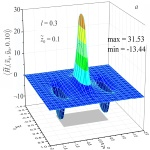## Observation of Brownian Relaxation of Magnetic Nanoparticles using HTS SQUID

### O. Snigirev$^{1,2}$, I. Soloviev$^3$, A. Kalabukhov$^4$, M. Chukharkin$^4$

Moscow University Physics Bulletin 2017. 72. N 1. P. 95

We analyzed the sensitivity of a separationless immunoassay scheme using functionalized magnetic nanoparticles (MNPs) and a sensitive HTS SQUID magnetometer. The signal of a 100 μL sample at a concentration of 1 mg/mL and field of 7.5 nT was 20 mΦ0. This makes it possible for the sensitivity to be within the range of 50 ng/mL at the required time of up to 100 s per a point in the frequency spectrum

Show Abstract